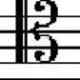# Diffraction Grating Spectrometry Question

1,225

So this was the answer given in the key:Share:
1,225Author by

### Mizuho

Updated on August 04, 2022

•Yes, this is a homework question, but I've already failed to solve it enough times that the online system hosting it isn't going to give me any marks, so I figure it's a good time to stop hitting the wall and actually ask. The question is as below:

A spectroscopist uses a spectrometer that has a grating with 600 grooves/mm. This grating can illuminate a CCD with a range of 1228 nm, in the 5th order (m = 5). One day, she buys a larger format CCD -- one larger than her old one by a factor of 3. What spectral range of wavelengths can be measured when she now obscures the 4th order lines (m = 4) with her larger CCD?

Came out with answers like $1.228 \times 10^{-6}$ and $4.17 \times 10^{-7}$ so far because I have no idea what the process is. Hints would suffice.

Basically, everything I've tried so far revolves around a formula: $m\lambda=d\sin\theta$.

What did I do so far:

• $5(1.228\times10^{-9})=\frac{0.001}{600}\times\sin\theta$ to get $\sin\theta$ and then put it back in to replace $\sin\theta$ as $\frac{4}{5}\sin\theta$. Then I went and searched up a diffraction grating to find out that the orders are not equidistant.
• $5(1.228\times10^{-9})=\frac{0.001}{600}\times\sin 90^\circ$ where I assumed the angle is perpendicular.
• Other stuff I can't remember

I feel like I understood the wavelength portion of this incorrectly, but I have no idea why.

•Brandon Enright over 8 years
Hi Mizuho, you need to describe what you've already tried and your basic thought process to have any hope of getting a good answer to your homework question.
•Bugasu over 8 years
A minor note, I don't know if it also existed in your calculations, but 1228 nm is $1.228 \times 10^{-6}$ NOT $1.228 \times 10^{-9}$
•Carl Witthoft over 8 years
Seems kind of odd: "grating can illuminate..." doesn't mean much to me. I suppose your teacher means the CCD is placed at a distance such that the 5th-order pattern fills N cm of space (the CCD width) with 1228nm spectral range, then at the same distance from the grating, place a CCD that is 3*N wide.
•dmckee --- ex-moderator kitten over 8 years
This being someone else's work it should probably have a proper attribution. Saying it was "given in the key" is a good start, but is not really complete. Who should get the credit for the text?